## Overview

• This article investigates over how the Vision Transformer (ViT) works by going over the minor modifications of the transformer architecture for image classification.

• We recommend checking out the articles on Transformer and attention if you don’t feel that comfortable with the terms.

• Transformers lack the inductive biases of Convolutional Neural Networks (CNNs), such as translation invariance and a locally restricted receptive field. You’ve probably heard that before. But what does it actually mean? Well, invariance means that you can recognize an entity (i.e. object) in an image, even when its appearance or position varies. Translation in computer vision implies that each image pixel has been moved by a fixed amount in a particular direction.
• Moreover, remember that convolution is a linear local operator. We see only the neighbor values as indicated by the kernel.
• On the other hand, the transformer is by design permutation invariant. The bad news is that it cannot process grid-structured data. We need sequences! To this end, we will convert a spatial non-sequential signal to a sequence! Let’s see how.

## How the Vision Transformer works in a nutshell

• The total architecture is called Vision Transformer (ViT), proposed by Alexey Dosovitskiy et al. (2020) in An Image is Worth 16x16 Words: Transformers for Image Recognition at Scale. Let’s examine it step by step.

1. Split an image into patches.
2. Flatten the patches.
3. Produce lower-dimensional linear embeddings from the flattened patches.
4. Add positional embeddings.
5. Feed the sequence as an input to a standard transformer encoder.
6. Pretrain the model with image labels (fully supervised on a huge dataset).
7. Finetune on the downstream dataset for image classification.
• The following image from Google’s AI blog shows the inner workings of ViT: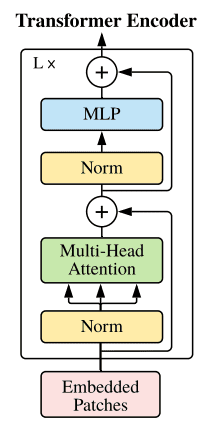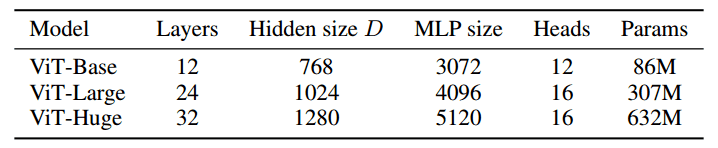• Heads refer to multi-head attention, while the MLP size refers to the blue module in the figure. MLP stands for multi-layer perceptron but it’s actually a bunch of linear transformation layers.

• Hidden size DD is the embedding size, which is kept fixed throughout the layers. Why keep it fixed? So that we can use short residual skip connections.

• In case you missed it, there is no decoder in the game. Just an extra linear layer for the final classification called MLP head. But is this enough? Yes and no. Actually, we need a massive amount of data and as a result computational resources.

## Important details

• Specifically, if ViT is trained on datasets with more than 14M images it can approach or beat state-of-the-art CNNs. If not, you better stick with ResNets or EfficientNets.

• ViT is pretrained on the large dataset and then fine-tuned to small ones. The only modification is to discard the prediction head (MLP head) and attach a new D \times KD×K linear layer, where K is the number of classes of the small dataset.

It is interesting that the authors claim that it is better to fine-tune at higher resolutions than pre-training.

• To fine-tune in higher resolutions, 2D interpolation of the pre-trained position embeddings is performed. The reason is that they model positional embeddings with trainable linear layers. Having that said, the key engineering part of this paper is all about feeding an image in the transformer.

## Representing an image as a sequence of patches

• Let’s go over how you can elegantly reshape the image in patches. For an input image $$(x) \in R^{H} \times W \times C$$ and patch size $$p$$, we want to create $N$ image patches denoted as $$(x) p \in R^{N} \times\left(P^{2} C\right)$$, where $$N=\frac{H W}{P} \cdot N$$ is the sequence length similar to the words of a sentence.
• If you didn’t notice the image patch i.e. [16,16,3] is flattened to 16x16x3. The title of the paper should now make sense :)
• Let’s use the einops library that works atop PyTorch. You can install it via pip:
\$ pip install einops

• And then some compact Pytorch code:
from einops import rearrange

p = patch_size # P in maths

x_p = rearrange(img, 'b c (h p1) (w p2) -> b (h w) (p1 p2 c)', p1 = p, p2 = p)

• In short, each symbol or each parenthesis indicates a dimension. For more information on einsum operations check out this blogpost on einsum operations.
• Note that the image patches are always squares for simplicity.
• And what about going from patch to embeddings? It’s just a linear transformation layer that takes a sequence of $$P^{2} C$$ elements and outputs $$D$$.
patch_dim = (patch_size**2) * channels # D in math

patch_to_embedding = nn.Linear(patch_dim, dim)

• What’s missing is that we need to provide some sort of order.

## Positional embeddings

• Even though many positional embedding schemes were applied, no significant difference was found. This is probably due to the fact that the transformer encoder operates on a patch-level. Learning embeddings that capture the order relationships between patches (spatial information) is not so crucial. It is relatively easier to understand the relationships between patches of $$P \times P$$ than of a full image $$Height \times Width$$.

Intuitively, you can imagine solving a puzzle of 100 pieces (patches) compared to 5000 pieces (pixels).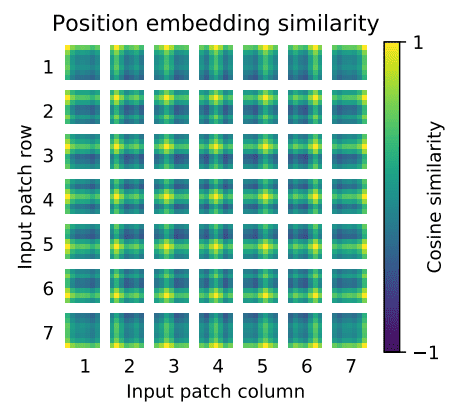• First, there is some kind of 2D structure. Second, patterns across rows (and columns) have similar representations. For high resolutions, a sinusoidal structure was used.

## Key findings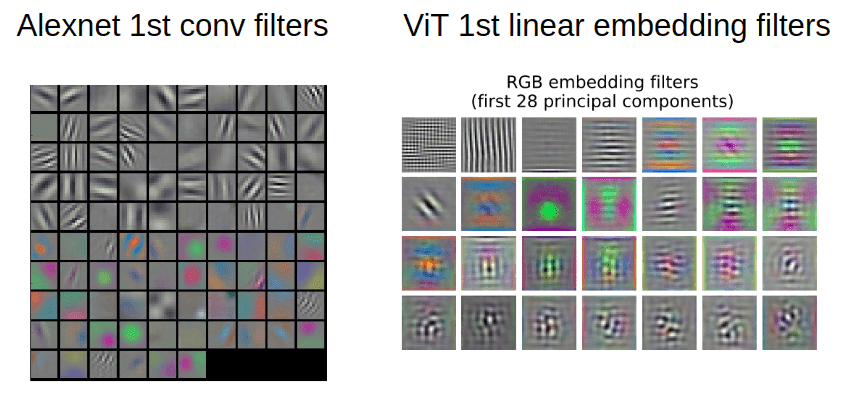• As stated in CS231n:

“Notice that the first-layer weights are very nice and smooth, indicating a nicely converged network. The color/grayscale features are clustered because the AlexNet contains two separate streams of processing, and an apparent consequence of this architecture is that one stream develops high-frequency grayscale features and the other low-frequency color features.” ~ Stanford CS231 Course: Visualizing what ConvNets learn

• For such visualizations PCA is used. In this way, the author showed that early layer representations may share similar features.

## How far aways are the learned non-local interactions?

• Short answer: For patch size $$P$$, maximum $$P*P$$, which in our case is 128, even from the 1st layer!

• We don’t need successive conv. layers to get to 128-away pixels anymore. With convolutions without dilation, the receptive field is increased linearly. Using self-attention we have interaction between pixels representations in the 1st layer and pairs of representations in the 2nd layer and so on. The following image shows the mean attention distance v/s the network depth (source: An Image is Worth 16x16 Words: Transformers for Image Recognition at Scale) the receptive field growth on the right (source: generated using Fomoro AI calculator).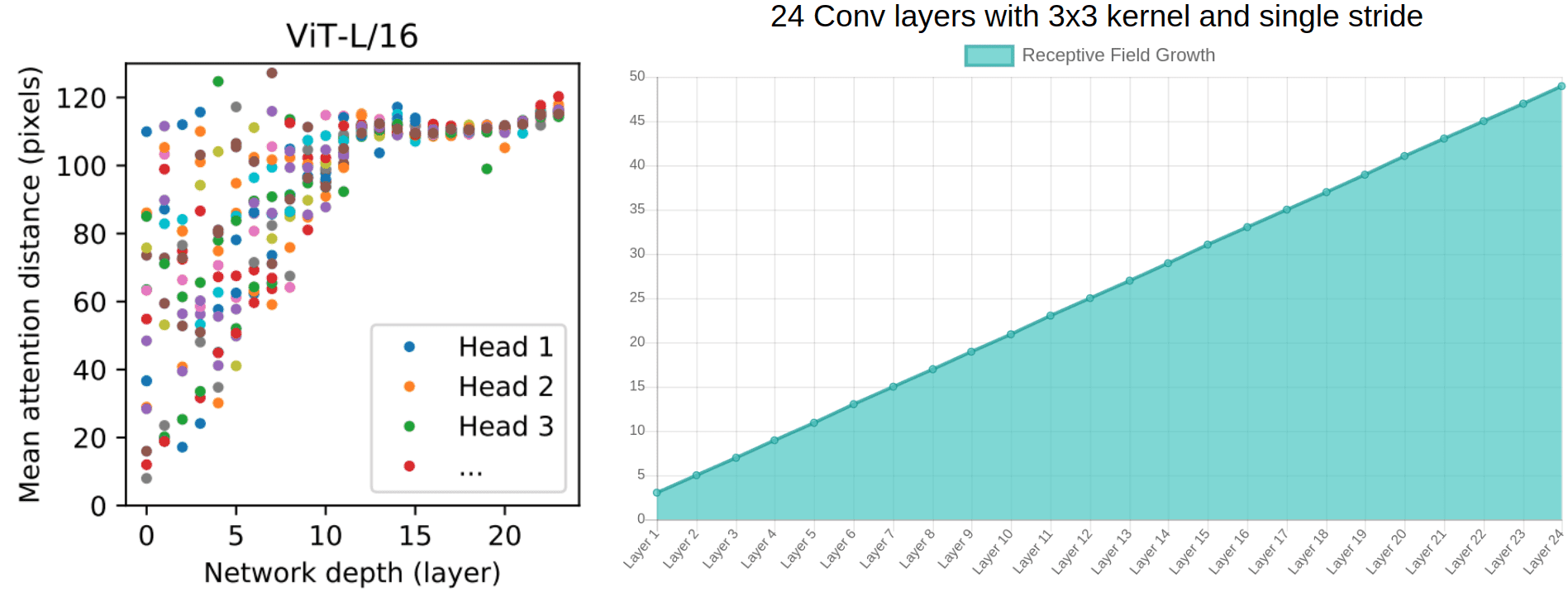• Based on the diagram on the left from ViT, one can argue that:
• There are indeed heads that attend to the whole patch already in the early layers.
• One can justify the performance gain based on the early access pixel interactions. It seems more critical for the early layers to have access to the whole patch (global info). In other words, the heads that belong to the upper left part of the image may be the core reason for superior performance.
• Interestingly, the attention distance increases with network depth similar to the receptive field of local operations.
• There are also attention heads with consistently small attention distances in the low layers. On the right, a 24-layer with standard 3x3 convolutions has a receptive field of less than 50. We would approximately need 50 conv layers, to attend to a ~100 receptive field, without dilation or pooling layers.
• To enforce this idea of highly localized attention heads, the authors experimented with hybrid models that apply a ResNet before the Transformer. They found less highly localized heads, as expected. Along with filter visualization, it suggests that it may serve a similar function as early convolutional layers in CNNs.

## Attention distance and visualization

• It is critical to understand how they measured the mean attention distance. It’s analogous to the receptive field, but not exactly the same.
• Attention distance was computed as the average distance between the query pixel and the rest of the patch, multiplied by the attention weight. They used 128 example images and averaged their results.
• An example: if a pixel is 20 pixels away and the attention weight is 0.5 the distance is 10.
• Finally, the model attends to image regions that are semantically relevant for classification, as illustrated below (source: An Image is Worth 16x16 Words: Transformers for Image Recognition at Scale):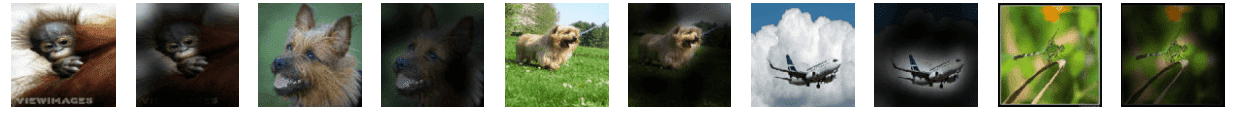## Implementation

• Given an implementation of the vanilla Transformer encoder, ViT looks as simple as this:
import torch
import torch.nn as nn
from einops import rearrange

from self_attention_cv import TransformerEncoder

class ViT(nn.Module):
def __init__(self, *,
img_dim,
in_channels=3,
patch_dim=16,
num_classes=10,
dim=512,
blocks=6,
dim_linear_block=1024,
dropout=0, transformer=None, classification=True):
"""
Args:
img_dim: the spatial image size
in_channels: number of img channels
patch_dim: desired patch dim
num_classes: classification task classes
dim: the linear layer's dim to project the patches for MHSA
blocks: number of transformer blocks
dim_linear_block: inner dim of the transformer linear block
dim_head: dim head in case you want to define it. defaults to dim/heads
dropout: for pos emb and transformer
transformer: in case you want to provide another transformer implementation
classification: creates an extra CLS token
"""
super().__init__()
assert img_dim % patch_dim == 0, f'patch size {patch_dim} not divisible'
self.p = patch_dim
self.classification = classification
tokens = (img_dim // patch_dim) ** 2
self.token_dim = in_channels * (patch_dim ** 2)
self.dim = dim
self.project_patches = nn.Linear(self.token_dim, dim)

self.emb_dropout = nn.Dropout(dropout)
if self.classification:
self.cls_token = nn.Parameter(torch.randn(1, 1, dim))
self.pos_emb1D = nn.Parameter(torch.randn(tokens + 1, dim))
self.mlp_head = nn.Linear(dim, num_classes)
else:
self.pos_emb1D = nn.Parameter(torch.randn(tokens, dim))

if transformer is None:
dim_linear_block=dim_linear_block,
dropout=dropout)
else:
self.transformer = transformer

def expand_cls_to_batch(self, batch):
"""
Args:
batch: batch size
Returns: cls token expanded to the batch size
"""
return self.cls_token.expand([batch, -1, -1])

def forward(self, img, mask=None):
batch_size = img.shape
img_patches = rearrange(
img, 'b c (patch_x x) (patch_y y) -> b (x y) (patch_x patch_y c)',
patch_x=self.p, patch_y=self.p)
# project patches with linear layer + add pos emb
img_patches = self.project_patches(img_patches)

if self.classification:
img_patches = torch.cat(
(self.expand_cls_to_batch(batch_size), img_patches), dim=1)

patch_embeddings = self.emb_dropout(img_patches + self.pos_emb1D)

# feed patch_embeddings and output of transformer. shape: [batch, tokens, dim]
y = self.transformer(patch_embeddings, mask)

if self.classification:
# we index only the cls token for classification. nlp tricks :P
return self.mlp_head(y[:, 0, :])
else:
return y


## Conclusion

• The key engineering part of this work is the formulation of an image classification problem as a sequential problem by using image patches as tokens, and processing it by a Transformer. That sounds good and simple but it needs massive data. Unfortunately, Google owns the pretrained dataset so the results are not reproducible. And even if they were, you would need to have enough computing power.

## Citation

If you found our work useful, please cite it as:

@article{Chadha2020DistilledVisionLanguageModels,
title   = {Vision Language Models},
author  = {Chadha, Aman},
journal = {Distilled AI},
year    = {2020},
note    = {\url{https://aman.ai}}
}